# Can anyone help me with these problems please!!! College phyiscs, second part

1. Four point charges are at the corners of a square of side a as shown in the figure below. Determine the magnitude and direction of theresultant electric force on q, with ke, q, and a left in symbolic form. (B = 5.5q and C = 5q. Letthe +x-axis be pointing to the right.)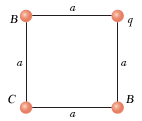A. F= N

B. Direction ° counter-clockwise from the +x-axis

2. Calculate the magnitude and direction of the Coulomb force on each of the three charges shown in the figure below.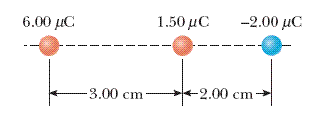6.00 µC charge

A. Magnitude N

B. Direction:towards the left
or
towardsthe right

1.50 µC charge

A. Magnitude N

B. Direction:towards the left
or
towards the right

-2.00 µC charge

A. Magnitude N

B. Direction:towards the left
or
towards the right

3.The figure below shows the electric field lines for two point charges separated by a small distance.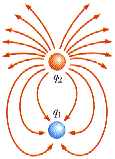(a) Determine the ratio q1/q2.

(b) What are the signs of q1 and q2?

q1 is positive. _____
q1 is negative.
____
q2 is positive.____

q2 is negative. ____

4. A 8.2 nC charge is located 2.1 m from a 3.5 nC charge. Find the magnitude of the electrostatic force that one charge exerts on the other.

a.

b. Is the force attractive or repulsive?

5. Four closed surfaces, S1 through S4, together with the charges -2Q, Q, and –Q are sketched in the figure below. Thecolored lines are the intersections of the surfaces with the screen.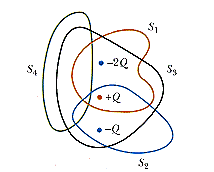Find the electric flux through each surface. (Use any variable or symbol stated above along with the following as necessary:e0.)

Fthrough S1 = ________
Fthrough S2 = ________

Fthrough S3 =_________

Fthrough S4 = ________

6. Suppose the conducting spherical shell of the figure below carries a charge of 3.10 nC and that a charge of -1.80 nC is at the center of thesphere. If a = 1.80 m and b = 2.30 m, find the electric field at the following.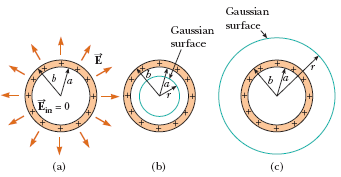(a) r = 1.50 m

_______N/C

(b) r = 2.20 m

_______N/C

(c) r = 2.50 m.
_______N/C

(d) What is the charge distribution on the sphere?

inner surface____N/C
outer surface____N/C

7.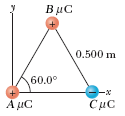(a) Three point charges, A = 2.2 µC, B = 7.5 µC, and C = -3.9 µC, are located at the corners of an equilateral triangle asin the figure above. Find the magnitude and direction of the electric field at the position of the 2.2 µC charge.

I. Magnitude____ N/C

II. Direction____ ° counter-clockwise from the +x-axis

(b) How would the electric field at that point be affected if the charge there were doubled?

A.___ The magnitude of the field would be halved.
B.___ The field would be unchanged.
C.____ The magnitude of the field woulddouble.
D.____ The magnitude of the field would quadruple.

(c) Would the magnitude of the electric force be affected?

___ no
___yes